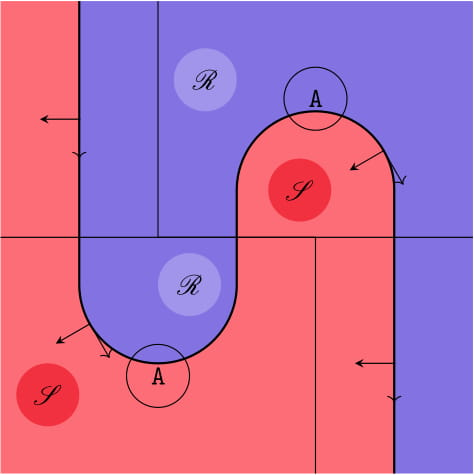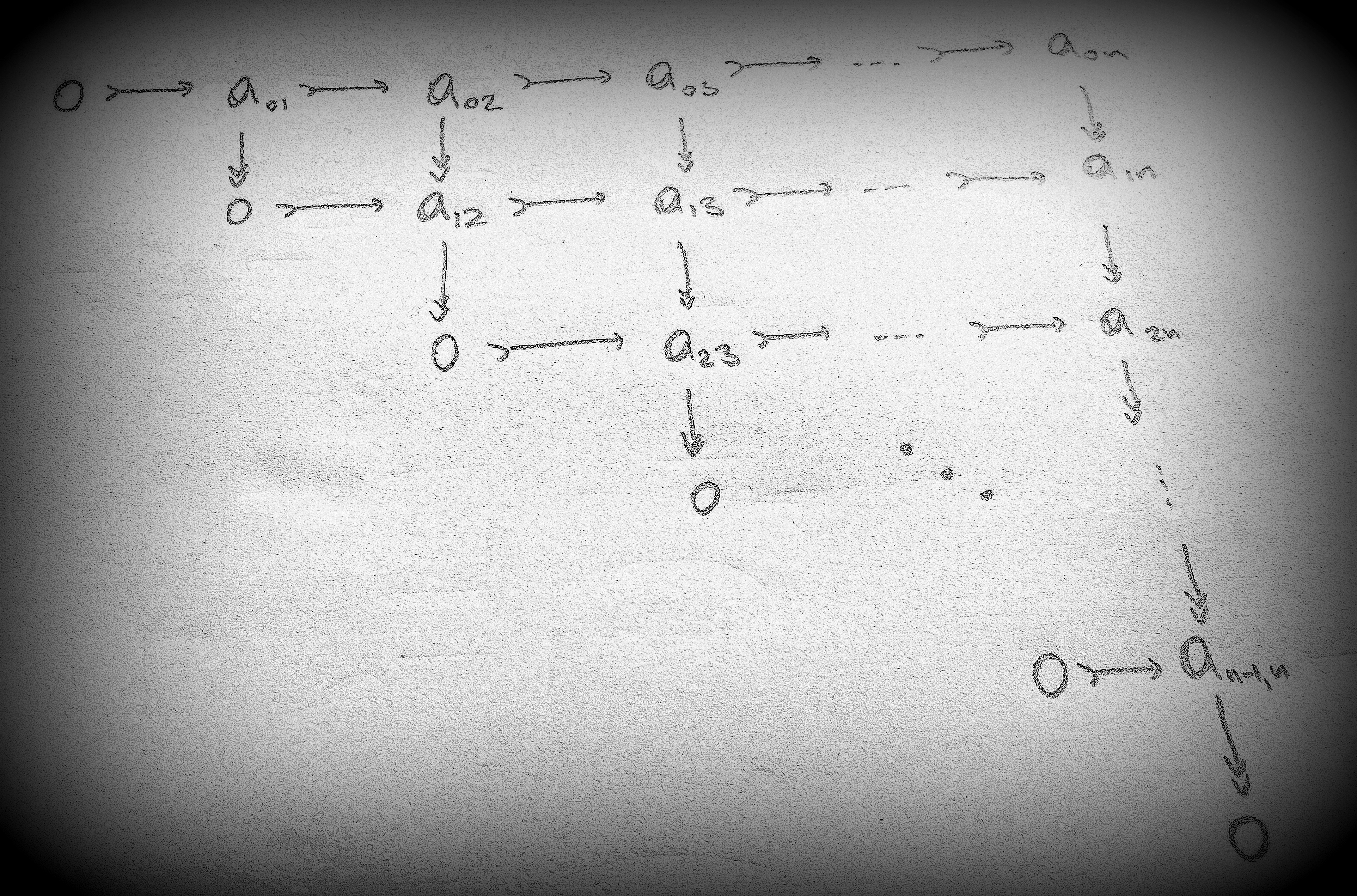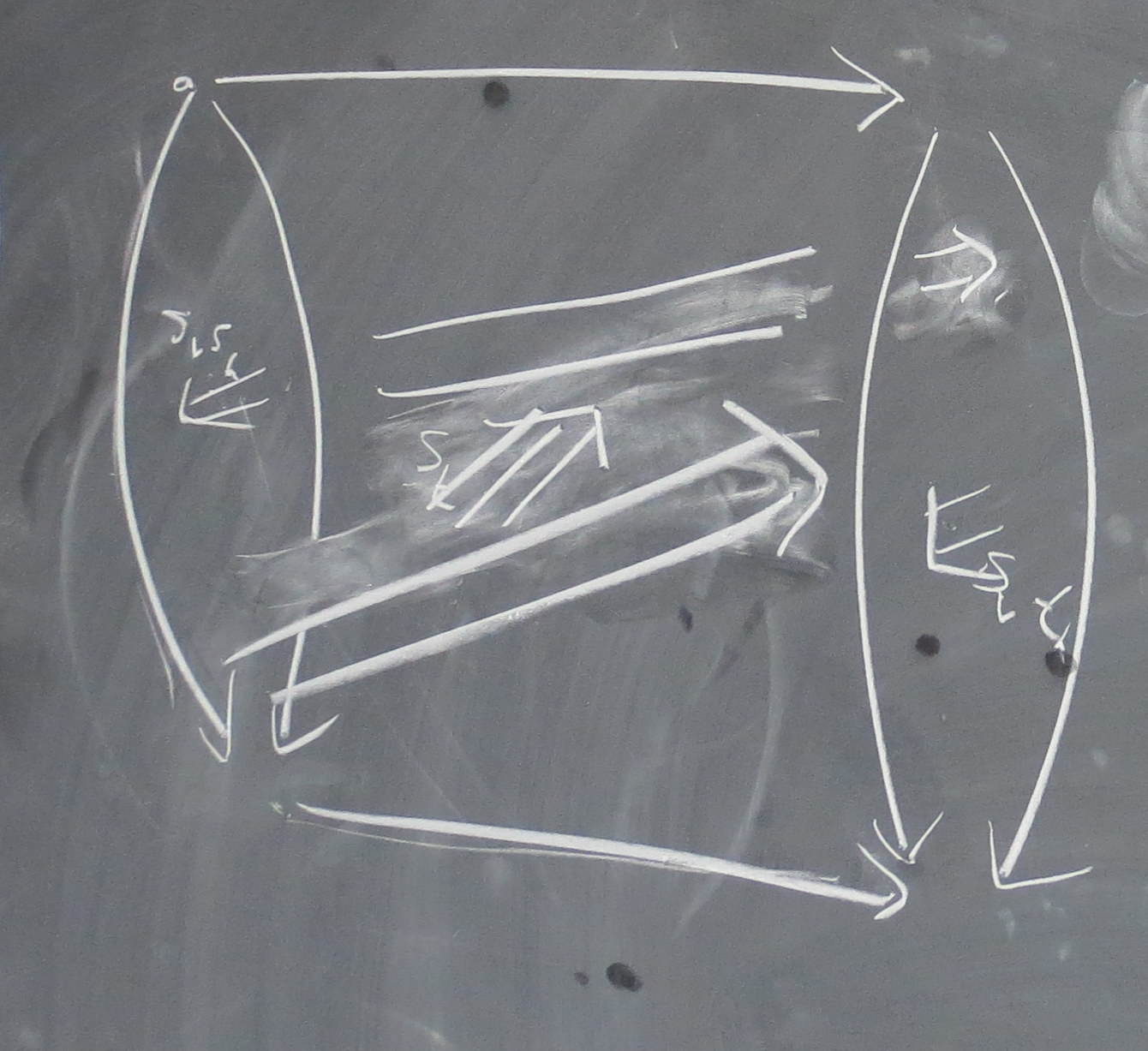Claudia I. Scheimbauer### research interests

My research area is mathematical physics. I work on fully extended topological field theories (in the sense of Lurie) using higher category theory, factorization algebras/homology, and derived symplectic geometry. I am also interested in open-closed field theories in the sense of Costello and Lurie and relative, or twisted, field theories in the sense of Freed-Teleman and Stolz-Teichner. Recently, I have become interested in questions revolving around objects carrying a higher categorical structure called "2-Segal object" which was introduced by Dyckerhoff-Kapranov and Gàlvez-Carrillo-Kock-Tonks and generalizes the idea of a categorical structure with a multivalued composition. These questions include applications in topology (Waldhausen construction and K-theory, configuration spaces) and mathematical physics (modular functors).

### articles

The AKSZ construction in derived algebraic geometry as an extended topological quantum field theory arxiv:2108.02473
with Damien Calaque and Rune Haugseng

We combine tools from extended topological field theories, derived algebraic geometry and higher categories to prove that the AKSZ construction of shifted symplectic forms on σ-models, i.e.~derived mapping stacks extends to a fully extended semi-classical TFT. This extends work by Pantev—Toën—Vaquié—Vezzosi and Calaque. The construction is two fold: on the one hand, they show full dualizability and use the Cobordism Hypothesis; on the other hand, they exhibit the symmetric monoidal functor in question, thus demonstrating the Cobordism Hypothesis in action. Examples include classical Chern–Simons theory, classical Rozansky–Witten theory, and the Poisson sigma model.

Comparison of Waldhausen constructions arxiv:1901.03606
with Julia E. Bergner, Angélica M. Osorno, Viktoriya Ozornova, and Martina Rovelli

We generalize our previous, discrete, result to the homotopical setting, by showing that there is a Quillen equivalence between a model category for unital 2-Segal objects and a model category for augmented stable double Segal objects which is given by an S-construction.

2-Segal objects and the Waldhausen construction arxiv:1809.10924
with Julia E. Bergner, Angélica M. Osorno, Viktoriya Ozornova, and Martina Rovelli

We generalize our previous, discrete, result to the homotopical setting, by showing that there is a Quillen equivalence between a model category for unital 2-Segal objects and a model category for augmented stable double Segal objects which is given by an S-construction.

The edgewise subdivision criterion for 2-Segal objects, accepted for publication in Proceedings of the AMS, arxiv:1807.05069
with Julia E. Bergner, Angélica M. Osorno, Viktoriya Ozornova, and Martina Rovelli

We show that the edgewise subdivision of a 2-Segal object is always a Segal object, and furthermore that this property characterizes 2-Segal objects.

Duals and adjoints in the factorization higher Morita category arxiv:1804.10924
with Owen Gwilliam

We study dualizability in the factorization (∞,n+N)-Morita category Alg_n(S) of E_n-algebras, bimodules in E_{n-1}-algebras, bimodules of bimodules, etc. in an (∞,N)-category S. More precisely, we that the symmetric monoidal (∞,n)-category underlying Alg_n(S) is fully n-dualizable, i.e. every object has a dual and every k-morphism has a left and a right adjoint for 0 < k < n. The Cobordism Hypothesis gives an immediate application of this result, namely the existence of categorified n-dimensional topological field theories (which were constructed explicitly in my PhD thesis using factorization homology, see below), and relative versions thereof. The motivation for this result was to construct low-dimensional examples of relative, "twisted", field theories in a subsequent article.

2-Segal sets and the Waldhausen construction, Topology and its Applications 23 (2018) pp. 445-484, arxiv:1609.02853
with Julia E. Bergner, Angélica M. Osorno, Viktoriya Ozornova, and Martina Rovelli

We give a variant of Waldhausen's S-construction which allows to take in certain double categories and showed that this is an equivalence of categories to the category of multivalued categories (unital 2-Segal sets). An extension of this construction to the homotopical setting should appear soon in a follow-up project.

(Op)lax natural transformations, twisted quantum field theories, and "even higher" Morita categories, Advances in Mathematics 307 (2017) pp. 147-223, arxiv:1502.06526
with Theo Johnson-Freyd

The main motivation for this project was to give a precise definition of twisted field theories in the setting of higher categories. This led to the development of a framework for lax and oplax transformations and their higher analogs between strong (∞,n)-functors. Another motivation was to give a construction of the higher Morita category of E_d-algebras in a symmetric monoidal (∞,n)-category C as an (∞,n+d)-category, thus extending the construction of my thesis below. Examples include the (∞,4)-category of braided monoidal (nice, linear) categories, monoidal bimodule categories, bimodule categories, functors, and natural transformations.

A note on the (∞,n)-category of cobordisms, Algebr. Geom. Topol., 19(2):533–655, 2019, arxiv:1509.08906
with Damien Calaque

Inspired by Lurie's paper on the cobordism hypothesis we define an n-fold Segal space of cobordisms, which is a good model for the (∞,n)-category of cobordisms.

Lectures on mathematical aspects of (twisted) supersymmetric gauge theories, Mathematical Aspects of Quantum Field Theories, Damien Calaque and Thomas Strobl, editors, Mathematical Physics Studies. Springer International Publishing, 2015, arxiv:1401.2676
with Kevin Costello

### thesis

#### Factorization homology as a fully extended topological field theory

We first give a precise definition of a fully extended n-dimensional topological field theory using complete n-fold Segal spaces as a model for (∞,n)-categories and then, given an E_n-algebra A, we explicitly construct a fully extended TFT given by taking factorization homology with coefficients in A. This is the fully extended n-TFT corresponding (via the cobordism hypothesis) to the E_n-algebra A, which is a fully dualizable object in a suitable Morita-(∞,n)-category Alg_n of E_n -algebras.

Some notes from my talk at the Winter School in Mathematical Physics 2014 in Les Diablerets. A video of a talk I gave about my thesis is available here.

Here is the current version of my thesis on "Factorization Homology as a Fully Extended Topological Field Theory".